## Operator Blob_Analysis_2D

The Blob_Analysis_2D operator detects objects in binary images and determines their properties. The outputs of the operator are several streams of data which represent properties for each object.

The functionality of the operator can be fully simulated in VisualApplets. However, please note that the order of the output values might not be equal to the hardware implementation.

Library License Required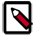Please note that you need a special license for the VisualApplets Blob Library if you want to use this operator.

Make sure you first read the general introduction into the blob analysis operators (section Library Blob).

### Performance

The VisualApplets blob analysis operators belong to the few operators whose processing speed, i.e., bandwidth, depends on the image content. Are many objects in the image, a large object list is created. This may result in slowing down the operator input. However, this applies only for images containing very strong noise. For controlled conditions, the operator should be sufficiently fast.

### Latency

The operator works with minimum latency. Input images are transferred to the blob analysis line by line. As soon as an object has been transferred into the operator completely, the operator detects object completion and outputs the object features. Thus, post-processing of object features can be started while the image itself is still being processed by the operator.

Note that the DMA and some other operators wait for the frame end signal before they report completion. The frame end signal is generated once the input image is finalized.

### Input Ports

The ImageI input of the blob analysis is represented by a binary, two-dimensional image, that is, an image having a bit width of 1 Bit, a specified image width, and a specified image height. You can select a parallelism of up to 32 pixels.

The operator assumes foreground values to have value ONE, and background values to have value ZERO. Make sure you match this requirement in the preliminary binarization process.

### Output Ports

Each of the output ports represents one object feature / object property. Each output value at these ports represents one object. Hence, each object property results in one data stream. The length of the output data streams is equal to the number of objects found in the image. The streams can be interrupted into several sub-frames. The data on each output port has a height of 1 pixel and a specific length. The output is represented in form of grayscale 2D images.

The output ports are configured using the operator parameters and properties of the input. The direct change of a link property is not possible.

The port ErrorFlagsO outputs several error flags of overflows. Each bit is reserved for a special flag. A detailed explanation can be found in the parameter description below. A summary is given in the following table:

Bit # Description Object Related Notes
0, 1 label overflow no

The Blob Analysis has detected too many objects to store in memory. To increase the maximum number of objects within two image lines, operator parameter "LabelBits" can be changed.

The flag is set upon detection until the end of the data output frame. All object properties which have been output so far are valid.

For the Blob Analysis 1D operator, the flag has only high-level state for one clock cycle together with the next valid data output.

After the flag is set, the output of the blob analysis might result in wrong object properties. The operator returns to correct functionality if enough object labels are available.

2 output truncated yes

The number of objects in the image exceed the maximum output width set in the operator parameters dialog. The flag is set together with the last object which fits into the output width.

3 object size exceeds maximum yes

The flag is set if an object of the 1D Blob Analysis exceeds its maximum height. The operator will cut this exceeding object into smaller objects. The continuative object is marked with the flag to allow the detection of a cut.

For 2D Blob Analysis the flag is set to const zero.

4 area is truncated yes

The area of the object together with the error flag is larger than the area bits allow.

5 center of gravity X is truncated yes

The center of gravity in X-direction is larger than the bits allow.

6 center of gravity Y is truncated yes

The center of gravity in Y-direction is larger than the bits allow.

7 contour length overflow yes

The contour length is larger than the bits parameterized for the operator allow.

Table 26. Explanation of Blob Error Flags

### I/O Properties

Property Value
Operator Type M
BoundingBoxX1O, data output
BoundingBoxY0O, data output
BoundingBoxY1O, data output
AreaO, data output
CenterXO, data output
CenterYO, data output
ContourOrthoO, data output
ContourDiaO, data output
ErrorFlagsO, data output

Bit Width 1 auto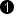auto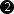auto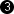auto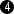auto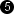auto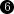auto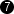auto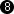auto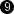8
Arithmetic unsigned unsigned unsigned unsigned unsigned unsigned unsigned unsigned unsigned unsigned unsigned
Parallelism {1, 2, 4, 8, 16, 32} 1 1 1 1 1 1 1 1 1 1
Kernel Columns 1 1 1 1 1 1 1 1 1 1 1
Kernel Rows 1 1 1 1 1 1 1 1 1 1 1
Img Protocol VALT_IMAGE2D VALT_IMAGE2D VALT_IMAGE2D VALT_IMAGE2D VALT_IMAGE2D VALT_IMAGE2D VALT_IMAGE2D VALT_IMAGE2D VALT_IMAGE2D VALT_IMAGE2D VALT_IMAGE2D
Color Format VAF_GRAY VAF_GRAY VAF_GRAY VAF_GRAY VAF_GRAY VAF_GRAY VAF_GRAY VAF_GRAY VAF_GRAY VAF_GRAY VAF_GRAY
Color Flavor FL_NONE FL_NONE FL_NONE FL_NONE FL_NONE FL_NONE FL_NONE FL_NONE FL_NONE FL_NONE FL_NONE
Max. Img Width any auto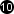auto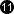auto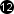auto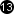auto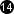auto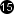auto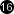auto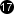auto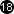auto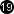Max. Img Height any 1 1 1 1 1 1 1 1 1 1
 The output bit width depends on the parameter settings of the object feature. If a feature is disabled, the output is set to one bit which is always value zero. The output maximum image width depends on the settings of parameter output_frame_size.

### Parameters

label_bits
Type static parameter
Default 7
Range [5, 31]

This parameter sets the number of bits which are used to label the objects internally. It also influences the maximum number of objects which may coexist within two image lines determined by 2^label_bits. Note that the required memory resources for the blob analysis almost double with every additional bit. A good value range for this parameter is between 7 and 9 bits which should be sufficient for almost every application.

If an overflow of this address space is detected, the operator outputs error flags at bits 0 and 1 of port "ErrorFlagsO". These flags are set upon detection of the overflow and are reset with the end of the output frame.

neighborhood
Type static parameter
Default eight_connected
Range {four_connected, eight_connected}

Select the required neighborhood for object detection. See 'Definition' for a detailed explanation of pixel neighborhoods.

output_frame_size
Type static parameter
Default specify_max_no_of_objects
Range {maximum_required, specify_max_no_of_objects}

The maximum number of objects which may theoretically populate an image is: Input image width * input image height / 2 (equal to a check pattern using 4-connected neighborhood). This pattern is quite unlikely. Therefore, you can configure the maximum number of objects in the images via this parameter.

max_output_frame_size
Type static parameter
Default 65536
Range [1, 67108864]

Select the maximum number of objects at the output. Any more objects detected by the blob analysis will be discarded. However, on port "ErrorFlagsO", an error flag at bit 2 will be set. The flag is set together with the last valid object. This parameter is disabled if "ouput_frame_size" is set to "maximum_required".

bounding_box_x0
Type static parameter
Default used
Range {used, not_used}

A bounding box represents the minimum paraxial rectangle which fits over the object. Each coordinate value is relatively to the top left corner of the image. The bounding box X0 represents the lowest x-coordinate of each object. It is output at "BoundingX0O". The bit width is determined from the input image. If the bounding box is not used, the bit width is set to one bit with constant zero at its output.

bounding_box_x1
Type static parameter
Default used
Range {used, not_used}

A bounding box represents the minimum paraxial rectangle which fits over the object. Each coordinate value is relatively to the top left corner of the image. The bounding box X1 represents the highest x-coordinate of each object. It is output at "BoundingX1O". The bit width is determined from the input image. If the bounding box is not used, the bit width is set to one bit with constant zero at its output.

bounding_box_y0
Type static parameter
Default used
Range {used, not_used}

A bounding box represents the minimum paraxial rectangle which fits over the object. Each coordinate value is relatively to the top left corner of the image. The bounding box Y0 represents the lowest y-coordinate of each object. It is output at "BoundingY0O". The bit width is determined from the input image. If the bounding box is not used, the bit width is set to one with constant zero at its output.

bounding_box_y1
Type static parameter
Default used
Range {used, not_used}

A bounding box represents the minimum paraxial rectangle which fits over the object. Each coordinate value is relatively to the top left corner of the image. The bounding box Y1 represents the highest y-coordinate of each object. It is output at "BoundingY1O". The bit width is determined from the input image. If the bounding box is not used, the bit width is set to one with constant zero at its output.

area_mode
Type static parameter
Default use_area_with_maximum_required_bits
Range {area_not_used, use_area_with_maximum_required_bits, use_area_with_specified_bits}

The area of an object is defined by the sum of all object foreground pixels. This parameter is used to select the required area mode. If "use_area_with_maximum_required_bits" is selected, the operator will automatically determine the required bits for the maximally possible size of an object. The maximally possible size of an object dependeds on the maximum width and height at the input link I. The theoretical maximum of an object is achieved if all pixels of an image consist of foreground values, i.e., a white input image which is one large object.

If users can be sure that their objects will not have a larger area than a specified value, this can be parameterized. Select "use_area_with_specified_bits" if the maximum object size is known. Use parameter "area_bits" to specify the number of bits. If the area is not required at all, select "area_not_used". In "area_not_used" mode, the bit width is set to one with constant zero at its output.

area_bits
Type static parameter
Default not_used
Range {not_used, used}

This parameter is enabled only if parameter "area_mode" is set to "use_area_with_specified_bits". If the area of an object is larger than the selected bits allow for, the blob analysis will output an overflow flag at bit 4 of the output link "ErrorFlagsO".

center_of_gravity_x_mode
Type static parameter
Default use_cX_with_maximum_required_bits
Range {cX_not_used, use_cX_with_maximum_required_bits, use_cX_with_specified_bits}

See 'Center of Gravity' for an explantion of the Center of Gravity.

Note that the output has to be divided by the area after blob analysis to get correct results.

If the parameter is set to "use_cX_width_maximum_required_bits", the operator will determine the output bits automatically. The required number of bits can get very high if large input images are used.

The number of bits is adjustable if "use_cX_with_specified_bits" is used for parameter value.

If no center of gravity in x-direction is required, it can be switched off by selecting "cX_not_used".

center_of_gravity_x_bits
Type static parameter
Default 29
Range [2, auto]

If "use_cX_with_specified_bits" is set for "center_of_gravity_x_mode", the bits can be changed here. Otherwise, the parameter is disabled.

center_of_gravity_x_overflow_flag
Type static parameter
Default not_used
Range {not_used, used}

If the number of bits for the center of gravity in x-direction is set manually, this parameter is activated and can be set to "used". The operator will then output a ONE at bit index 5 of the output link "ErrorFlagsO" if an overflow is detected.

center_of_gravity_y_mode
Type static parameter
Default use_cY_with_maximum_required_bits
Range {cY_not_used, use_cY_with_maximum_required_bits, use_cY_with_specified_bits}

See 'Center of Gravity' for an explantion of the Center of Gravity.

Note that the output has to be divided by the area after blob analysis to get correct results.

If the parameter is set to "use_cY_width_maximum_required_bits", the operator will determine the output bits automatically. The required number of bits can get very high if large input images are used.

The number of bits is adjustable if "use_cY_with_specified_bits" is used for parameter value.

If no center of gravity in y-direction is required, it can be switched off by selecting "cY_not_used".

center_of_gravity_y_bits
Type static parameter
Default 29
Range [2, auto]

If "use_cY_with_specified_bits" is set for "center_of_gravity_y_mode", the bits can be changed here. Otherwise, the parameter is disabled.

center_of_gravity_y_overflow_flag
Type static parameter
Default not_used
Range {not_used, used}

If the number of bits for the center of gravity in y-direction is set manually, this parameter is activated and can be set to "used". The operator will then output a ONE at bit index 6 of the output link "ErrorFlagsO" if an overflow is detected.

contour_length_mode
Type static parameter
Default contour_length_not_used
Range {contour_length_not_used, contour_length_used}

The contour length of an object includes all edges, even at holes. For a detailed explanation see 'Contour Length'.

If this feature is selected, the required bits can be chosen with the parameters "contour_length_bits_orthogonal_connected" and "contour_length_bits_diagonal_connected" depending on the selected neighborhood. If a 4-connected neighborhood is selected, the contour length can only be determined in orthogonal directions. If an 8-connected neighborhood is selected, the contour length is determined in orthogonal and diagonal directions.

contour_length_bits_orthogonal_connected
Type static parameter
Default 16
Range [1, 31]

If "contour_length_mode" is set to "contour_length_used", the bits required to represent the contour length for orthogonally connected pixels can be chosen here.

contour_length_bits_diagonal_connected
Type static parameter
Default 16
Range [1, 31]

If "contour_length_mode" is set to "contour_length_used", the bits required to represent the contour length for diagonally connected pixels can be chosen here. This is only possible if an 8-connceted neighborhood is selected. Otherwise this parameter is disabled.

contour_length_overflow_flag
Type static parameter
Default not_used
Range {not_used, used}

The contour length may cause an overflow. If the flag is used, an error flag is set at bit number 7 of port "ErrorFlagsO" in case of an overflow.

### Examples of Use

The use of operator Blob_Analysis_2D is shown in the following examples: